Johnson-Neyman图通常简称为J-N图， 主要用于可视化调节效应。

# J-N图已有方案的缺陷

• 最著名的是 SAS 和 SPSS 的 PROCESS 插件（Hayes，2013b）。这有一些问题， 一些研究人员无法使用 SAS 或 SPSS，因为这些软件的价格昂贵。
• SPSS绘图很丑，JN 图形输出需要“大量编辑”（Hayes，2013b，第 242 页）。
• 可以肯定的是，有免费的解决方案，但它们至少存在以下缺点之一：图形质量差，流程不够精简，需要用户事先完成线性回归，然后才能进行JN分析

# 技术细节

Y代表因变量， X是自变量， M是调节变量。

$Ŷ=\left({\stackrel{^}{\gamma }}_{0}+{\stackrel{^}{\gamma }}_{2}M\right)+\left({\stackrel{^}{\gamma }}_{1}+{\stackrel{^}{\gamma }}_{3}M\right)X.$

$Ŷ={\stackrel{^}{\omega }}_{0}\left(M\right)+{\stackrel{^}{\omega }}_{1}\left(M\right)X.$

${SE}_{{\stackrel{^}{\omega }}_{1}\left(M\right)}=\sqrt{\mathrm{\text{Var}}\left({\stackrel{^}{\gamma }}_{1}\right)+2M\mathrm{\text{Cov}}\left({\stackrel{^}{\gamma }}_{1},{\stackrel{^}{\gamma }}_{3}\right)+{M}^{2}\mathrm{\text{Var}}\left({\stackrel{^}{\gamma }}_{3}\right)}.$

$t=\frac{{\stackrel{^}{\omega }}_{1}\left(M\right)}{{SE}_{{\stackrel{^}{\omega }}_{1}\left(M\right)}}$

# Excel绘制JN图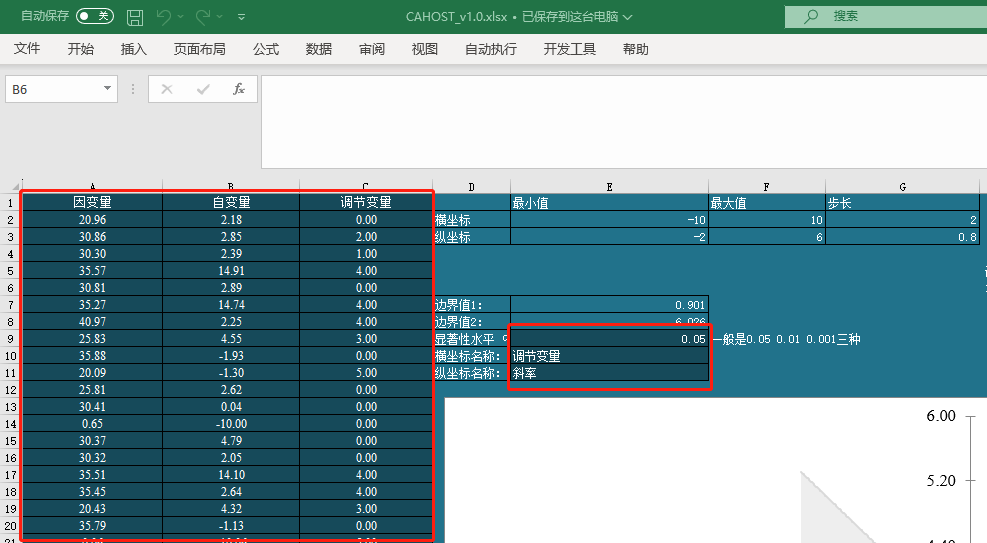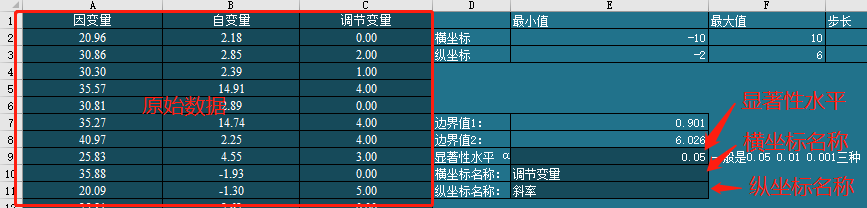• 首先，我们注意表格中输出了坐标轴的一些建议参数，你可以参考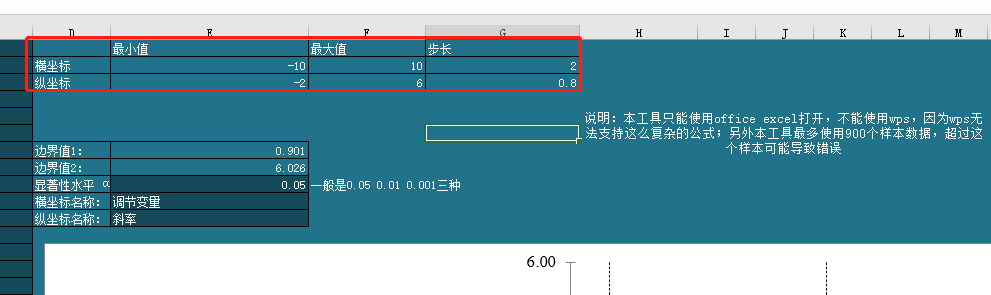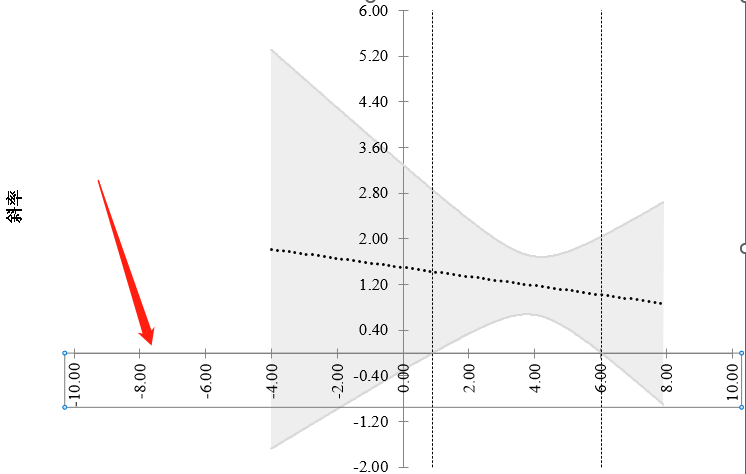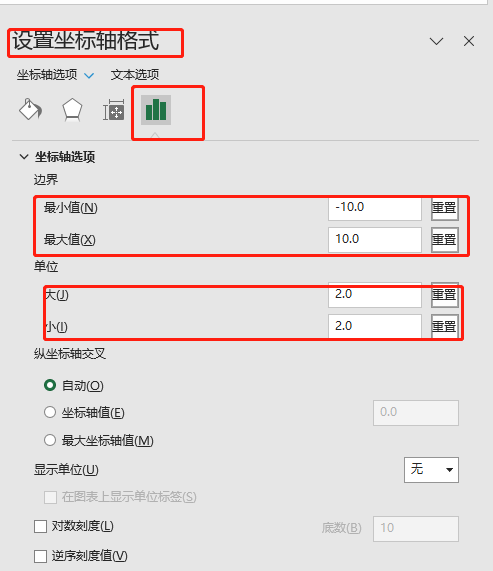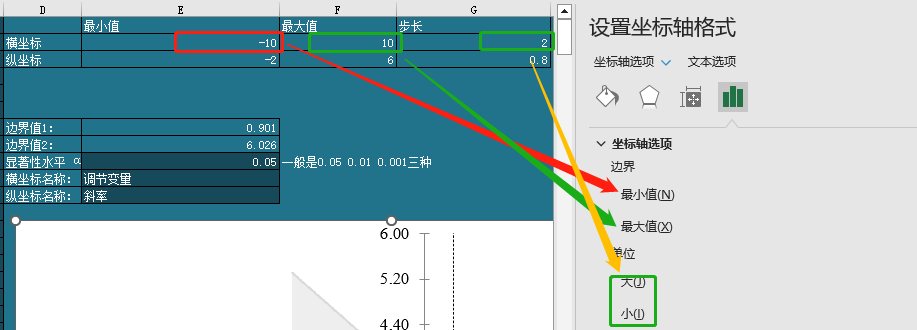# Johnson-Neyman图如何解读

• 案例1： 当调节变量M的取值小于-1.7的时候，斜率是显著的；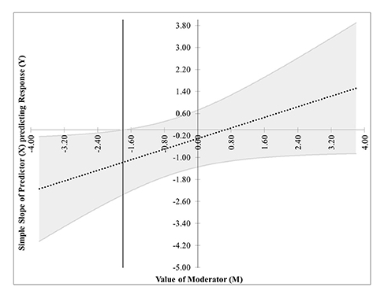• 案例2： 当调节变量M的取值大于1.5的时候，斜率是显著的；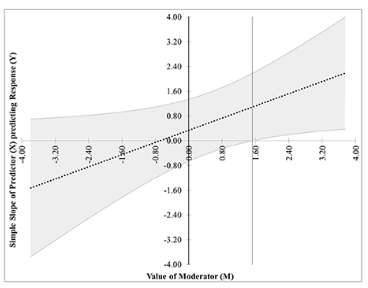• 案例3： 当调节变量在1到6之间的时候，斜率是显著的；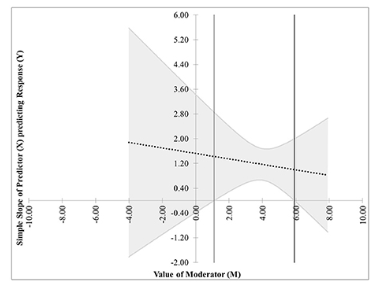• 案例4： 当调节变量小于0.1或者大于4.8的时候，斜率是显著的；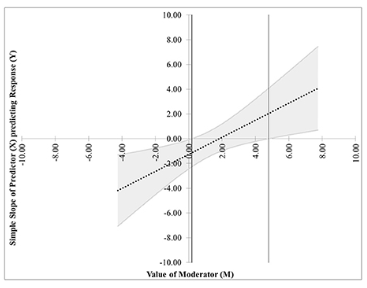• 案例5： 调节变量的所有取值范围下，斜率都是显著的；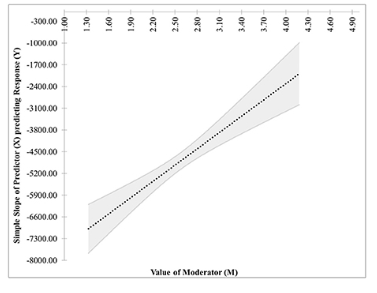• 案例6： 调节变量的所有取值范围下， 斜率都是不显著的；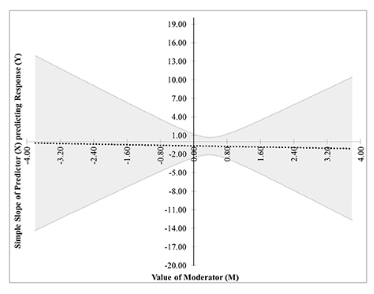# 工具从哪里下载

### 赞助